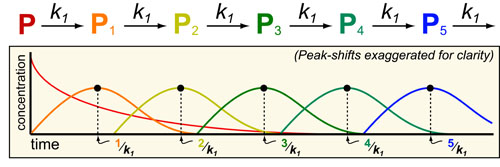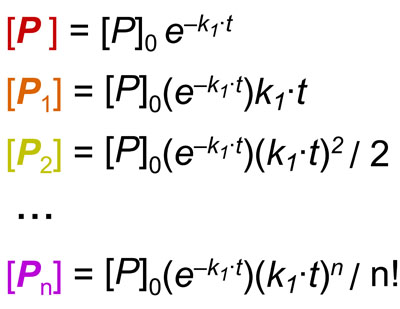# Kinetics #6: Pulse-Chase ExperimentsIn our sixth post on Understanding Kinetics, we consider pulse-chase experiments which are a common method to study bio-synthetic pathways. In pulse-chase experiments:

1. A “pulse” of labeled metabolite (P) is added to culture media.
2. The down-stream intermediates ((P1, P2, P3, etc.)) are measured (“chase“) with mass-spectrometry or radiation.

Examples of common pulse-chase experiments include:

• using heavy-glucose to study glycolysis
• using heavy-glutamine to study glutaminolysis
• using heavy-acetate to study fatty acid synthesis

Assuming pseudo-first order kinetics, bio-synthetic pathways are generally analytically solvable as detailed reference 1. Unfortunately, these models can become rather complex as the pathways become larger which can make it difficult to get an intuitive feel for the dynamics of such processes. If one further assumes that each step in the pathway has a similar psuedo-first order rate (a somewhat reasonable assumption as large rate differences would cause inefficiency in the “assembly-line”) the mathematics simplifies dramatically for any sequential pathway to the equations below (where k1 is the pseudo-first order rate constant and Pn is the nth intermediate) :Based on the equations above, in general, the pulse of each nth intermediate will occur at approximately n/k1.

### REFERENCES:

1. Banford, C.H.; Tipper, C.F.H. Comprehensive Chemical Kinetics. Elsevier, 1969
2. Segel, I.H. Enzyme Kinetics: Behavior and Analysis of Rapid Equilibrium and Steady-State Enzyme Systems, Wiley-Interscience, 1993
3. Lauffenburger, D.A. Receptors: Models for Binding, Trafficking and Signalling, Oxford University Press 1993.